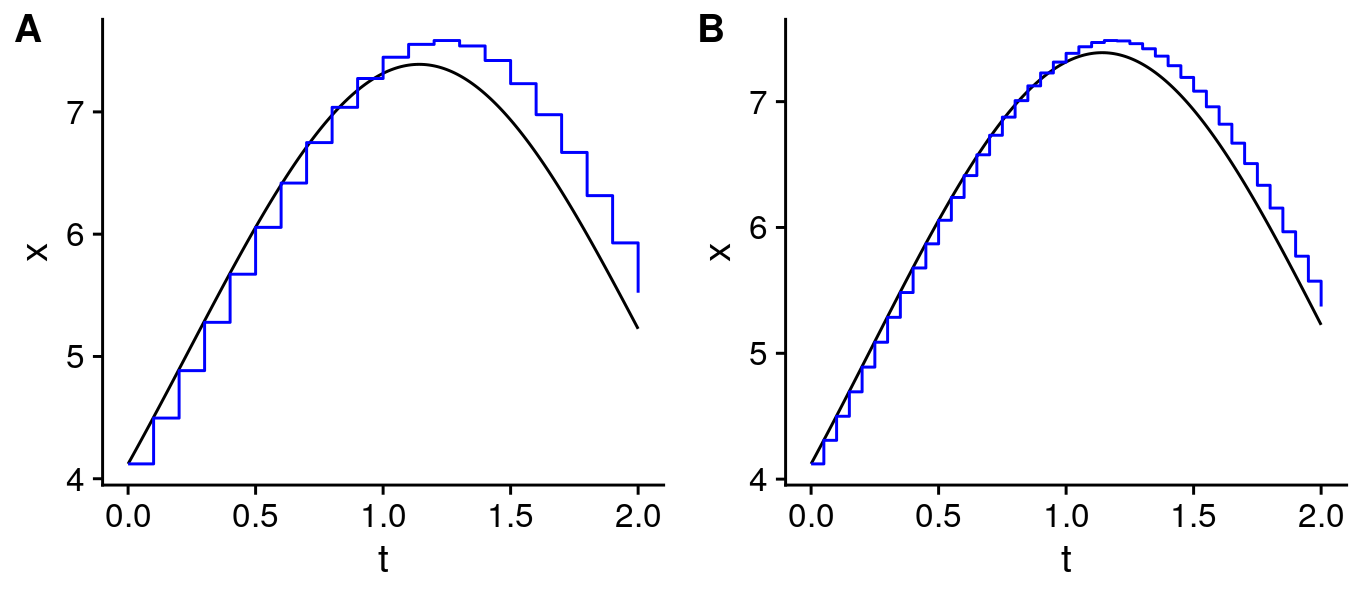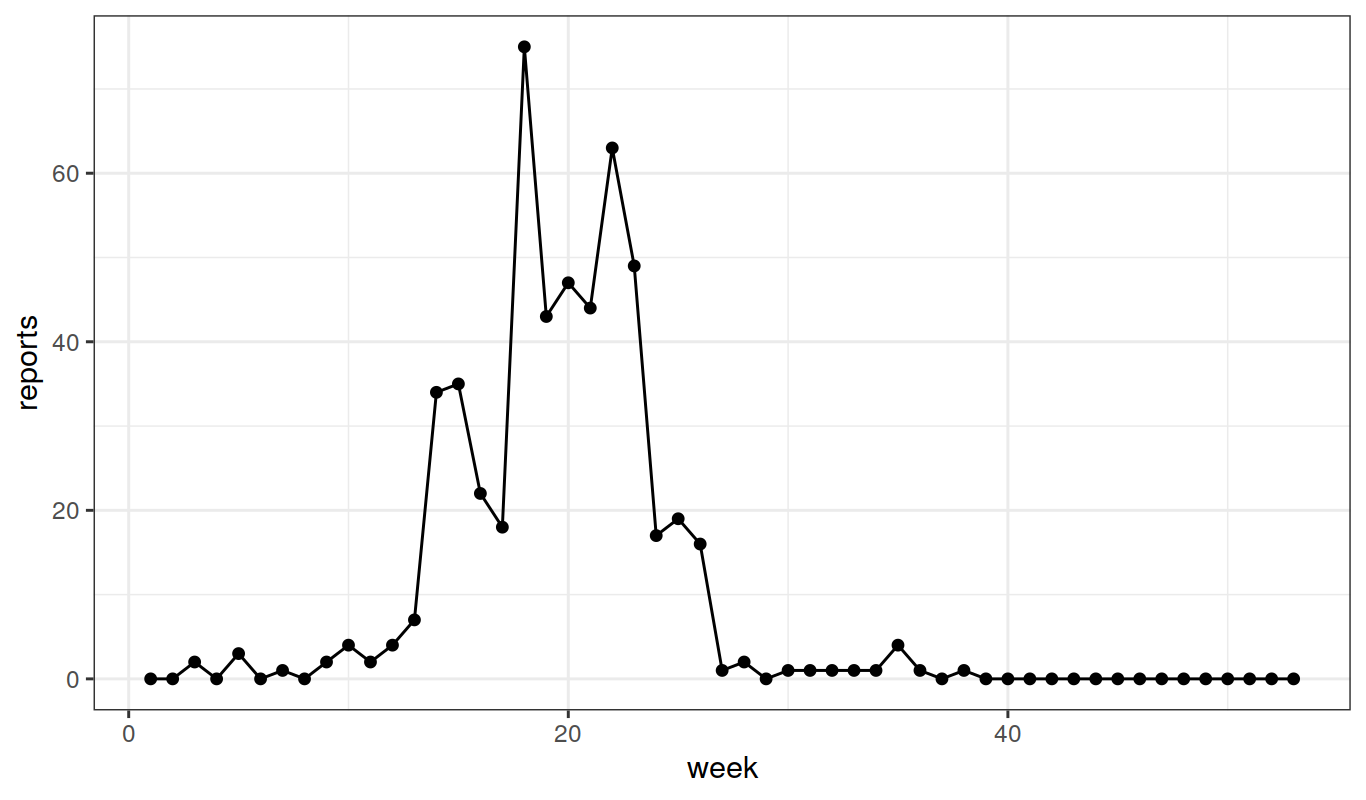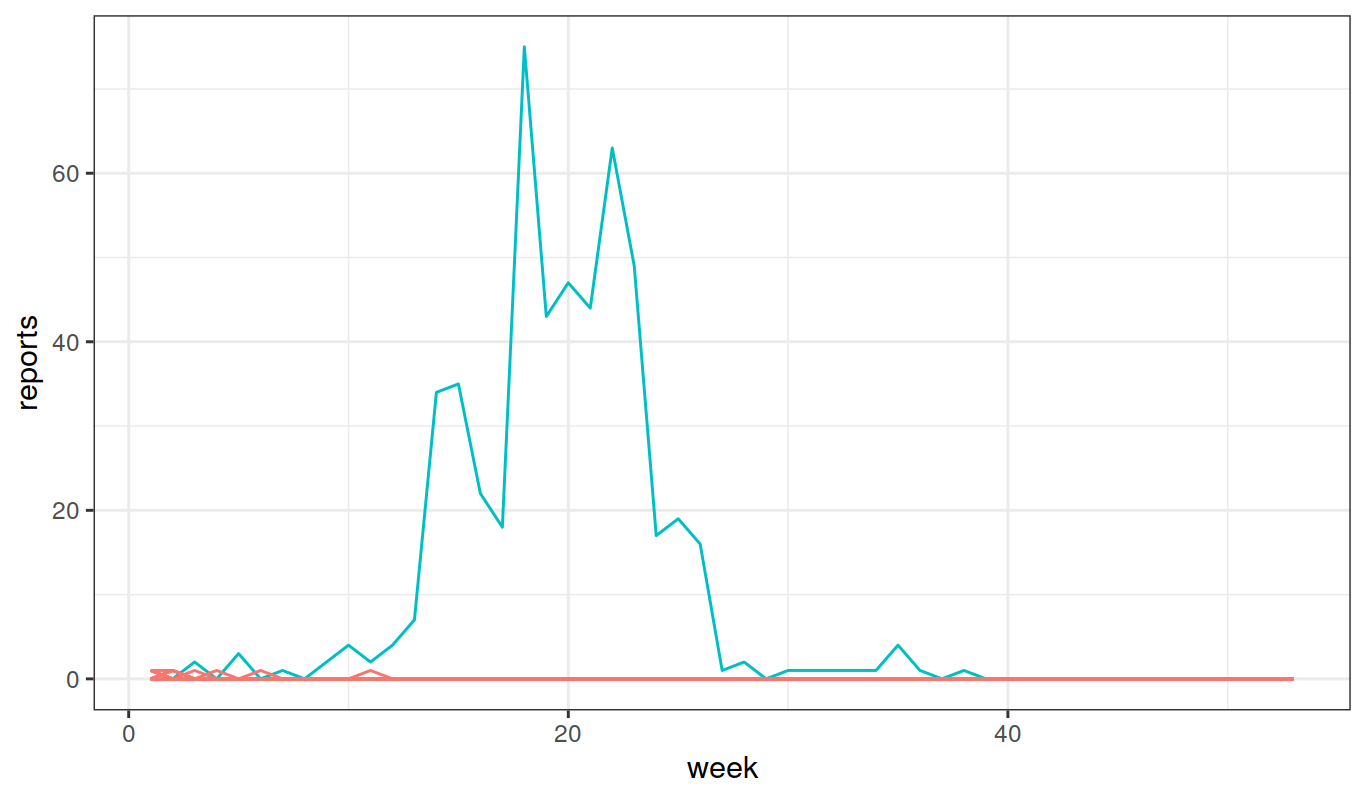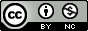## Objectives

This tutorial develops some classes of dynamic models of relevance in biological systems, especially epidemiology. We have the following goals:

1. Dynamic systems can often be represented in terms of flows between compartments. We will develop the concept of a compartmental model for which we specify rates for the flows between compartments.
2. We show how deterministic and stochastic versions of a compartmental model are derived and related.
3. We introduce Euler’s method to simulate from dynamic models.
4. We specify deterministic and stochastic compartmental models in pomp using Euler method simulation.

## Introduction

• Compartmental models are of great utility in many disciplines and very much so in epidemiology.

• We start by deriving deterministic and stochastic versions of the susceptible-infected-recovered (SIR) model of disease transmission dynamics in a closed population.

• In so doing, we develop notation that generalizes to systems of greater complexity (Bretó et al. 2009).Diagram of the SIR compartmental model.

• Let $$S(t)$$, $$I(t)$$, and $$R(t)$$ represent, respectively, the number of susceptible hosts, the number of infected (and, by assumption, infectious) hosts, and the number of recovered or removed hosts. This corresponds to placing individuals into one of the compartments S, I and R in the diagram.

• We suppose that each arrow has an associated per capita rate, so here there is a rate $$\mu_{SI}$$ at which each individual in S transitions to I, and $$\mu_{IR}$$ at which each individual in I transitions to R.

• To account for demography (birth/death/migration) we allow the possibility of a source and sink compartment, which is not represented on the flow diagram above.

• We write $$\mu_{{\small\bullet} S}$$ for a rate of births into S.

• Mortality rates are denoted by $$\mu_{S{\small\bullet}}$$, $$\mu_{I{\small\bullet}}$$, $$\mu_{R{\small\bullet}}$$.

• The rates may be either constant or varying. In particular, for a simple SIR model, the recovery rate $$\mu_{IR}$$ is a constant but the infection rate has the time-varying form $\mu_{SI}(t)=\beta\,\frac{I(t)}{N(t)},$ with $$\beta$$ being the contact rate and $$N$$ the total size of the host population. In the present case, since the population is closed, we set $\mu_{{\small\bullet} S}=\mu_{S{\small\bullet}}=\mu_{I{\small\bullet}}=\mu_{R{\small\bullet}}=0.$

• In general, it turns out to be convenient to keep track of the flows between compartments as well as the number of individuals in each compartment.

• Let $$N_{SI}(t)$$ count the number of individuals who have transitioned from $$S$$ to $$I$$ by time $$t$$.

• We say that $$N_{SI}(t)$$ is a counting process.

• A similarly constructed process $$N_{IR}(t)$$ counts individuals transitioning from I to R.

• To include demography, we could keep track of birth and death events by the counting processes $$N_{{\small\bullet} S}(t)$$, $$N_{S{\small\bullet}}(t)$$, $$N_{I{\small\bullet}}(t)$$, $$N_{R{\small\bullet}}(t)$$.

• For discrete population compartment models, the flow counting processes are non-decreasing and integer valued.

• For continuous population compartment models, the flow counting processes are non-decreasing and real valued.

• The number of hosts in each compartment can be computed via these counting processes. Ignoring demography, we have: \begin{aligned} S(t) &= S(0) - N_{SI}(t)\\ I(t) &= I(0) + N_{SI}(t) - N_{IR}(t)\\ R(t) &= R(0) + N_{IR}(t)\\ \end{aligned}

• These equations represent a kind of conservation law. Over any finite time interval $$[t,t+\delta)$$, we have \begin{aligned} {\Delta}{S} &= -{\Delta}{N}_{SI}\\ {\Delta}{I} &= \phantom{-}{\Delta}{N}_{SI}-{\Delta}{N}_{IR}\\ {\Delta}{R} &= \phantom{-}{\Delta}{N}_{IR}, \end{aligned} where the $$\Delta$$ notation indicates the increment in the corresponding process.

• Thus, for example $${\Delta}{N}_{SI}(t) = N_{SI}(t+\delta)-N_{SI}(t)$$.

## Compartmental models as deterministic or stochastic Markov models.

• We suppose that flow rates between compartments depend only on the current state of the system. In this case, a compartmental model is Markovian.

• The deterministic, continuous time, continuous population version of a compartmental model is given by the solution to a system of coupled ordinary differential equation (ODEs).

• We will see various ways to include stochasticity (i.e., noise) in a compartmental model.

• Removing noise from a stochastic model to obtain an ODE model gives a deterministic skeleton of the stochastic model.

### The deterministic version of the SIR model

• Together with initial conditions specifying $$S(0)$$, $$I(0)$$ and $$R(0)$$, we just need to write down ordinary differential equations (ODE) for the flow counting processes. These are, $\begin{gathered} \frac{dN_{SI}}{dt} = \mu_{SI}(t)\,S(t), \qquad \frac{dN_{IR}}{dt} = \mu_{IR}\,I(t). \end{gathered}$

• Given initial values, all the future states in the model are exactly specified. This is suitable for highly predictable systems.

### The simple continuous-time Markov chain version of the SIR model

• Continuous-time Markov chains are the basic tool for building discrete population epidemic models.

• Recall that a Markov chain is a discrete-valued stochastic process with the Markov property: the future evolution of the process depends only on the current state.

• Surprisingly many models have this Markov property. If all important variables are included in the state of the system, then the Markov property appears automatically.

• The Markov property lets us specify a model by giving the transition probabilities on small intervals together with initial conditions.

• For the SIR model in a closed population, we have \begin{aligned} &\mathbb{P}\left[{N_{SI}(t+\delta)=N_{SI}(t)+1}\right] &=& &\mu_{SI}(t)\,S(t)\,\delta + o(\delta)\\ &\mathbb{P}\left[{N_{SI}(t+\delta)=N_{SI}(t)}\right] &=& &1-\mu_{SI}(t)\,S(t)\,\delta + o(\delta)\\ &\mathbb{P}\left[{N_{IR}(t+\delta)=N_{IR}(t)+1}\right] &=& &\mu_{IR}(t)\,I(t)\,\delta + o(\delta)\\ &\mathbb{P}\left[{N_{IR}(t+\delta)=N_{IR}(t)}\right] &=& &1-\mu_{IR}(t)\,I(t)\,\delta + o(\delta)\\ \end{aligned}

• A simple counting process is one for which no more than one event can occur at a time (Wikipedia: point process).

• Thus, in a technical sense, the SIR Markov chain model we have written is simple.

• One may want to model the extra randomness resulting from multiple simultaneous events: someone sneezing in a crowded bus, large gatherings at football matches, etc. This extra randomness may even be critical to match the variability in data.

• We will see later, in the measles case study, a situation where this extra randomness plays an important role. The representation of the model in terms of counting processes turns out to be useful for this.

#### Optional Exercise: From Markov chain to ODE

Find the expected value of $$N_{SI}(t+\delta)-N_{SI}(t)$$ and $$N_{IR}(t+\delta)-N_{IR}(t)$$ given the current state, $$S(t)$$, $$I(t)$$ and $$R(t)$$. Take the limit as $$\delta\to 0$$ and show that this gives the ODE model.

Worked solution to the Optional Exercise

## Euler’s method for numerical solution of dynamic models

Euler took the following approach to numeric solution of an ODE:

• He wanted to investigate an ODE $$\frac{dx}{dt}=h(x,t)$$ with an initial condition $$x(t_0)=x_0$$.

• Since, in many cases of interest, the true solution $$x(t)$$ of this ODE cannot be worked out analytically, Euler wished to find a numerical approximation, $$\tilde x(t)$$, to $$x(t)$$.

• He divided time into small intervals of length $$\delta$$, with $$\tilde t_k=t_0+k\,\delta$$.

• He initialized the numerical approximation at the known starting value, $\tilde x(t_0)=x(t_0)=x_0.$

• He then reasoned that the gradient, $$dx/dt$$, is roughly constant over each small time interval $$\tilde t_k\le t\le \tilde t_{k+1}$$, for $$k=1,2,\dots$$.

• Accordingly, he defined $\tilde{x}(\tilde t_{k+1}) = \tilde{x}(\tilde t_k) + \delta\,h\big(\tilde{x}(\tilde t_{k}),\tilde t_{k}).$

• This defines $$\tilde x(t)$$ only for $$t=\tilde t_{k}$$, but let’s suppose $$\tilde x(t)$$ is constant between these discrete times (see the figure below).

• We now have a numerical scheme stepping forward in time increments of size $$\delta$$ which can be readily evaluated by computer.

• Mathematical analysis of Euler’s method says that, as long as the function $$h(x)$$ is not too exotic, then $$x(t)$$ is well approximated by $$\tilde x(t)$$ when the discretization time-step, $$\delta$$, is sufficiently small.

• Euler’s method is not the only numerical scheme to solve ODEs. More advanced schemes have better convergence properties, meaning that the numerical approximation is closer to $$x(t)$$.

• There are 3 reasons we choose to lean heavily on Euler’s method:

1. Euler’s method is the simplest (the KISS principle).

2. Euler’s method extends naturally to stochastic models, both continuous-time Markov chains models and stochastic differential equation (SDE) models.

3. In the context of data analysis, close approximation of the numerical solutions to a continuous-time model is less important than may be supposed, a topic discussed later.Euler approximations $$x=\tilde x(t)$$ with stepsize 0.1 (A) and 0.05 (B) are shown in blue. The true solution, $$x=x(t)$$, is shown in black.

### Euler’s method for a discrete-valued SIR model

• Recall the simple continuous-time Markov chain interpretation of the SIR model without demography: \begin{aligned} \mathbb{P}\left[{N_{SI}(t+\delta)=N_{SI}(t)+1}\right] &= \mu_{SI}(t)\,S(t)\,\delta + o(\delta),\\ \mathbb{P}\left[{N_{IR}(t+\delta)=N_{IR}(t)+1}\right] &= \mu_{IR}\,I(t)\,\delta + o(\delta). \end{aligned}

• We look for a numerical solution with state variables $$\tilde S(t_k)$$, $$\tilde I(t_k)$$, $$\tilde R(t_k)$$.

• The counting processes for the flows between compartments are $$\tilde N_{SI}(t)$$ and $$\tilde N_{IR}(t)$$. The counting processes are related to the numbers of individuals in the compartments by the same flow equations we had before: \begin{aligned} {\Delta}{\tilde S} &= -{\Delta}{\tilde N}_{SI}\\ {\Delta}{\tilde I} &= {\Delta}{\tilde N}_{SI}-{\Delta}{\tilde N}_{IR}\\ {\Delta}{\tilde R} &= {\Delta}{\tilde N}_{IR}, \end{aligned}

• Let’s focus $$N_{SI}(t)$$; the same methods can also be applied to $$N_{IR}(t)$$.

• Three stochastic Euler schemes for $$N_{SI}$$ are enumerated below. Conceptually, it is simplest to think of schemes (1) and (2). Numerically, we prefer to use (3).

1. Poisson increments: ${\Delta}{\tilde N}_{SI}\;\sim\;\mathrm{Poisson}\left(\tilde \mu_{SI}(t)\,\tilde S(t)\,\delta\right),$ where $$\mathrm{Poisson}\left(\mu\right)$$ is the Poisson distribution with mean $$\mu$$ and $\tilde\mu_{SI}(t)=\beta\,\frac{\tilde I(t)}{N}.$

2. Binomial increments with linear probability: ${\Delta}{\tilde N}_{SI}\;\sim\;\mathrm{Binomial}\left(\tilde{S}(t),\tilde\mu_{SI}(t)\,\delta\right),$ where $$\mathrm{Binomial}\left(n,p\right)$$ is the binomial distribution with mean $$n\,p$$ and variance $$n\,p\,(1-p)$$.

3. Binomial increments with exponential decaying probability: ${\Delta}{\tilde{N}}_{SI}\;\sim\;\mathrm{Binomial}\left(\tilde{S}(t),1-e^{-\tilde{\mu}_{SI}(t)\,\delta}\right).$

• These schemes all become equivalent as $$\delta\to 0$$.

• Why is (3) usually advantageous in practice?

### Euler methods for stochastic differential equations (SDE) models

• A natural way to add stochastic variation to an ODE $$dx/dt=h(x)$$ is $\frac{dX}{dt}=h(X)+\sigma\,\frac{dB}{dt}$ where $$B(t)$$ is Brownian motion and so $$dB/dt$$ is Gaussian white noise.

• This is called a stochastic differential equation (SDE).

• The Euler method extends naturally to SDE models.

• The approximation $$\tilde X$$ is generated by $\tilde X(\tilde t_{k+1}) = \tilde X(\tilde t_k) + \delta\,h\big(\tilde X(\tilde t_k)\big) + \sigma \sqrt{\delta} \, Z_k$ where $$Z_1,Z_2,\dots$$ is a sequence of independent standard normal random variables, i.e., $$Z_k\sim\mathrm{Normal}\left(0,1\right)$$.

• SDEs are often considered an advanced topic in probability. However, working with SDE models specified by Euler solutions does not require advanced probability techniques. We only need familiarity with the normal distribution.

#### Optional Exercise: SDE version of the SIR model

Write down the Euler-Maruyama method for an SDE representation of the closed-population SIR model. Consider some difficulties that might arise with non-negativity constraints, and propose some practical way one might deal with that issue.

A useful method to deal with positivity constraints is to use Gamma noise rather than Brownian noise (Laneri et al. 2010, He et al. 2010, Bhadra et al. 2011). SDEs driven by Gamma noise can be investigated by Euler solutions simply by replacing the Gaussian noise by an appropriate Gamma distribution.

Worked solution to the Optional Exercise

### Euler’s method vs. Gillespie’s algorithm

• A widely used, exact simulation method for continuous time Markov chains is Gillespie’s algorithm (Gillespie 1977).

• We do not put much emphasis on Gillespie’s algorithm here. The Euler method is applicable to a wider class of models.

• When would you prefer an implementation of Gillespie’s algorithm to an Euler solution?

• Numerically, Gillespie’s algorithm is often approximated using so-called $$\tau$$-leaping methods (Gillespie 2001), which are closely related to Euler’s approach with $$\delta$$ being $$\tau$$.

• Indeed, an Euler solution for a continuous time Markov chain is sometimes called a Gillespie tau-leaping method.

### Some comments on discrete-time numerical solutions to dynamic systems

• In some physical situations, a system follows an ODE model closely. For example, Newton’s laws provide a very good approximation to the motions of celestial bodies.

• In many biological situations, ODE models become good approximations to reality only at relatively large scales. On small temporal scales, models cannot usually capture the full scope of biological variation and biological complexity.

• If we expect substantial error in using $$x(t)$$ to model a biological system, maybe the numerical solution $$\tilde x(t)$$ represents the biological system as well as $$x(t)$$ does.

• If our model fitting, model investigation, and final conclusions are all based on our numerical solution $$\tilde x(t)$$ (e.g., we are sticking entirely to simulation-based methods) then we are most immediately concerned with how well $$\tilde x(t)$$ describes the system of interest.

• $$\tilde x(t)$$ may be more important than the original model, $$x(t)$$.

• When following this perspective, it is important that one fully describe the numerical model $$\tilde x(t)$$.

• From this point of view, the role of the continuous-time model $$x(t)$$ is to provide a succinct way to describe how $$\tilde x(t)$$ was constructed.

• All numerical methods are, ultimately, discretizations.

• For continuous-time modeling, we usually aim to set $$\delta$$ small compared to the timescale of the process being modeled, so that the choice of $$\delta$$ does not play an explicit role in the interpretation of the model.

• Epidemiologically, setting $$\delta$$ to be a day, or an hour, can be quite different from setting $$\delta$$ to be two weeks or a month.

• Putting emphasis on the scientific role of the numerical solution is a reminder that the numerical solution has to do more than approximate a target model in a limit as $$\delta\to 0$$. The numerical solution should be a sensible model in its own right for the value of $$\delta$$ being used.

## Compartmental models in pomp.

### The Consett measles outbreak

• As an example that we can probe in some depth, let’s look at outbreak of measles that occurred in the small town of Consett in England in 1948.
read_csv("https://kingaa.github.io/sbied/stochsim/Measles_Consett_1948.csv") %>%
select(week,reports=cases) -> meas
as.data.frame(meas)### A simple POMP model for measles

• These are incidence data: The reports variable counts the number of reports of new measles cases each week.

• Let us model the outbreak using the simple SIR model.

• Our tasks will be, first, to estimate the parameters of the SIR and, second, to decide whether or not the SIR model is an adequate description of these data.

• Below is a diagram of the SIR model. The host population is divided into three classes according to their infection status: S, susceptible hosts; I, infected (and infectious) hosts; R, recovered and immune hosts.

• The rate at which individuals move from S to I is the force of infection, $$\mu_{SI}=\beta\,I/N$$, while that at which individuals move into the R class is $$\mu_{IR}$$.• Let’s look at how we can view the SIR as a POMP model.

• The unobserved state variables, in this case, are the numbers of individuals, $$S(t)$$, $$I(t)$$, $$R(t)$$ in the S, I, and R compartments, respectively.

• It’s reasonable in this case to view the population size $$N=S(t)+I(t)+R(t)$$, as fixed at the known population size of 38,000.

• The numbers that actually move from one compartment to another over any particular time interval are modeled as stochastic processes.

• In this case, we’ll assume that the stochasticity is purely demographic, i.e., that each individual in a compartment at any given time faces the same risk of exiting the compartment.

• Demographic stochasticity is the unavoidable randomness that arises from chance events occuring in a discrete and finite population.

### Implementing the model

• To implement the model in pomp, the first thing we need is a stochastic simulator for the unobserved state process.

• We’ve seen that there are several ways of approximating the process just described for numerical purposes.

• An attractive option here is to model the number moving from one compartment to the next over a very short time interval as a binomial random variable.

• In particular, we model the number, $${\Delta}{N_{SI}}$$, moving from S to I over interval $${\Delta}{t}$$ as ${\Delta}{N_{SI}} \sim \mathrm{Binomial}\left(S,1-e^{-\beta\,I/N{\Delta}{t}}\right),$ and the number moving from I to R as ${\Delta}{N_{IR}} \sim \mathrm{Binomial}\left(I,1-e^{-\mu_{IR}{\Delta}{t}}\right).$

sir_step <- function (S, I, R, N, Beta, mu_IR, delta.t, ...) {
dN_SI <- rbinom(n=1,size=S,prob=1-exp(-Beta*I/N*delta.t))
dN_IR <- rbinom(n=1,size=I,prob=1-exp(-mu_IR*delta.t))
S <- S - dN_SI
I <- I + dN_SI - dN_IR
R <- R + dN_IR
c(S = S, I = I, R = R)
}
• At day zero, we’ll assume that $$I=1$$ but we don’t know how many people are susceptible, so we’ll treat this fraction, $$\eta$$, as a parameter to be estimated.
sir_init <- function(N, eta, ...) {
c(S = round(N*eta), I = 1, R = round(N*(1-eta)))
}
• We fold these basic model components, with the data, into a pomp object thus:
meas %>%
pomp(times="week",t0=0,
rprocess=euler(sir_step,delta.t=1/6),
rinit=sir_init
) -> measSIR
• Now let’s assume that the case reports result from a process by which new infections are diagnosed and reported with probability $$\rho$$, which we can think of as the probability that a child’s parents take the child to the doctor, who recognizes measles and reports it to the authorities.

• Since measles symptoms tend to be quite recognizable, and children with measles tend to be confined to bed, diagnosed cases have, presumably, a much lower transmission rate. Accordingly, let’s treat each week’s reports as being related to the number of individuals who have moved from I to R over the course of that week.

• We need a variable to track these daily counts. Let’s modify our rprocess function above, adding a variable $$H$$ to tally the true incidence.

sir_step <- function (S, I, R, H, N, Beta, mu_IR, delta.t, ...) {
dN_SI <- rbinom(n=1,size=S,prob=1-exp(-Beta*I/N*delta.t))
dN_IR <- rbinom(n=1,size=I,prob=1-exp(-mu_IR*delta.t))
S <- S - dN_SI
I <- I + dN_SI - dN_IR
R <- R + dN_IR
H <- H + dN_IR;
c(S = S, I = I, R = R, H = H)
}

sir_init <- function (N, eta, ...) {
c(S = round(N*eta), I = 1, R = round(N*(1-eta)), H = 0)
}
• In pomp terminology, $$H$$ is an accumulator variable. Since we want $$H$$ to tally only the incidence over the week, we’ll need to reset it to zero at the beginning of each week. We accomplish this using the accumvars argument to pomp:
measSIR %>%
pomp(
rprocess=euler(sir_step,delta.t=1/6),
rinit=sir_init,accumvars="H"
) -> measSIR
• Now, we’ll model the data as a binomial process, $\mathrm{reports}_t \sim \mathrm{Binomial}\left(H(t)-H(t-1),\rho\right).$

• Now, to include the observations in the model, we must write either a dmeasure or an rmeasure component, or both:

dmeas <- function (reports, H, rho, log, ...) {
dbinom(x=reports, size=H, prob=rho, log=log)
}

rmeas <- function (H, rho, ...) {
c(reports=rbinom(n=1, size=H, prob=rho))
}
• We then put these into our pomp object:
measSIR %>% pomp(rmeasure=rmeas,dmeasure=dmeas) -> measSIR

### Specifying model components using C snippets

• Although we can always specify basic model components using R functions, as above, we’ll typically want the computational speed up that we can obtain only by using compiled native code.

• pomp provides a facility for doing so with ease, using C snippets.

• A C snippet is a small piece of C code used to specify a basic model component.

• For example, C snippets that encode the basic model components in sir are as follows.

sir_step <- Csnippet("
double dN_SI = rbinom(S,1-exp(-Beta*I/N*dt));
double dN_IR = rbinom(I,1-exp(-mu_IR*dt));
S -= dN_SI;
I += dN_SI - dN_IR;
R += dN_IR;
H += dN_IR;
")

sir_init <- Csnippet("
S = nearbyint(eta*N);
I = 1;
R = nearbyint((1-eta)*N);
H = 0;
")

dmeas <- Csnippet("
lik = dbinom(reports,H,rho,give_log);
")

rmeas <- Csnippet("
reports = rbinom(H,rho);
")
• A call to pomp replaces the basic model components with these, much faster, implementations:
measSIR %>%
pomp(rprocess=euler(sir_step,delta.t=1/6),
rinit=sir_init,
rmeasure=rmeas,
dmeasure=dmeas,
accumvars="H",
statenames=c("S","I","R","H"),
paramnames=c("Beta","mu_IR","N","eta","rho")
) -> measSIR
• Note that, when using C snippets, one has to tell pomp which of the variables referenced in the C snippets are state variables and which are parameters. This is accomplished using the statenames and paramnames arguments.

### Testing the model: simulations

• Let’s perform some simulations, just to verify that our codes are working as intended.

• To do so, we’ll need some parameters. A little thought will get us some ballpark estimates.

• For an SIR infection, one has that $$\mathfrak{R}_0\approx\frac{L}{A}$$, where $$L$$ is the lifespan of a host and $$A$$ is the mean age of infection. Analysis of age-stratified serology data establish that the mean age of infection for measles during this period was around 4–5yr (Anderson and May 1991). Assuming a lifespan of 60–70yr, we have a rough estimate of $$\mathfrak{R}_0\approx 15$$.

• From the basic theory of SIR epidemics, we have the final-size equation $\mathfrak{R}_0= -\frac{\log{(1-f)}}{f},$ where $$f$$ is the final size of the epidemic—the fraction of those susceptible at the beginning of the outbreak who ultimately become infected—and $$\mathfrak{R}_0$$ is the basic reproduction number. Recall that $$\mathfrak{R}_0$$ is the expected number of secondary infections resulting from one primary infection introduced into a fully susceptible population. For $$\mathfrak{R}_0>5$$, this equation predicts that $$f>0.99$$.

• In the data, it looks like there were a total of $$521$$ infections. Assuming 50% reporting, we have that $$S_0\approx1042$$, so that $$\eta=\frac{S_0}{N}\approx0.027$$.

• If the infectious period is roughly 2 wk, then $$1/\mu_{IR} \approx 2~\text{wk}$$ and $$\beta = \mu_{IR}\,\mathfrak{R}_0\approx 7.5~\text{wk}^{-1}$$.

• Let’s simulate the model at these parameters.

measSIR %>%
simulate(params=c(Beta=7.5,mu_IR=0.5,rho=0.5,eta=0.03,N=38000),
nsim=20,format="data.frame",include.data=TRUE) -> sims

sims %>%
ggplot(aes(x=week,y=reports,group=.id,color=.id=="data"))+
geom_line()+
guides(color=FALSE)Well, this leaves something to be desired. In the exercises, you’ll see if this model can do better.

### Exercises

#### Basic Exercise: Explore the SIR model

Fiddle with the parameters to see if you can’t find a model for which the data are a more plausible realization.

Worked solution to the Exercise

#### Basic Exercise: The SEIR model

Below is a diagram of the so-called SEIR model. This differs from the SIR model in that infected individuals must pass a period of latency before becoming infectious.Modify the codes above to construct a pomp object containing the flu data and an SEIR model. Perform simulations as above and adjust parameters to get a sense of whether improvement is possible by including a latent period.

Worked solution to the Exercise

## Acknowledgments

• This tutorial is prepared for the Simulation-based Inference for Epidemiological Dynamics module at 11th Annual Summer Institute in Statistics and Modeling in Infectious Diseases (SISMID 2019). Previous versions were presented at SISMID by ELI and AAK in 2015, 2016, 2017, 2018. Carles Breto assisted in 2018.